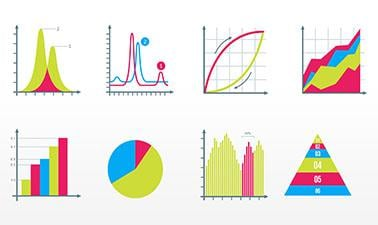# Probability and Statistics in Data Science using Python

Using Python, learn statistical and probabilistic approaches to understand and gain insights from data.After a course session ends, it will be archived.
Estimated 10 weeks
10–12 hours per week
Self-paced

The job of a data scientist is to glean knowledge from complex and noisy datasets.

Reasoning about uncertainty is inherent in the analysis of noisy data. Probability and Statistics provide the mathematical foundation for such reasoning.

In this course, part of the Data Science MicroMasters program, you will learn the foundations of probability and statistics. You will learn both the mathematical theory, and get a hands-on experience of applying this theory to actual data using Jupyter notebooks.

Concepts covered included: random variables, dependence, correlation, regression, PCA, entropy and MDL.

### At a glance

• Language: English
• Associated programs:

# What you'll learn

Skip What you'll learn
• The mathematical foundations for machine learning
• Statistics literacy: understand the meaning of statements such as "at a 99% confidence level"